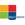Activities

••• Subject Area

• Math: Middle Grades Math: Statistics and Probability
• Math: PreAlgebra: Probability, Statistics and Data Analysis

• Author6-8

45 Minutes

• Device
• TI-83 Plus Family
• TI-84 Plus
• TI-84 Plus Silver Edition
• Other Materials
For more information on the Holt, Rinehart and Winston Mathematics Series, visit them on the web at www.hrw.com/math

Create Box-and-Whisker Plots

Activity Overview

Students use a graphing calculator to create box-and-whisker plots and learn the usefulness of them.

Students identify the five summary statistical values of data sets - minimum, first quartile, median, third quartile, and maximum values.

This Technology Lab accompanies Lesson 9-4 from the ©2007 Holt, Rinehart and Winston Mathematics Course 3 textbook.

Before the Activity

• Review the lesson PDF files.
• Make copies of the lesson PDF files for your class.
• During the Activity

Students use a graphing calculator to create box-and whisker plots and identify all five summary statistical values of data sets - minimum, first quartile, median, third quartile, and maximum values.

After the Activity

Review student results:

• As a class, discuss questions that appeared to be more challenging
• Re-teach concepts as necessary